### Home > MC2 > Chapter Ch7 > Lesson 7.1.6 > Problem7-60

7-60.
1. Forty percent of the students at Pinecrest Middle School have a school sweatshirt. There are 560 students at the school. Draw a diagram to help you solve each problem below. Homework Help ✎

1. How many students have a school sweatshirt?

2. If 280 students have school t-shirts instead of sweatshirts, what percentage of the school has a t-shirt?

3. What percentage of the school does not have a t-shirt or a sweatshirt?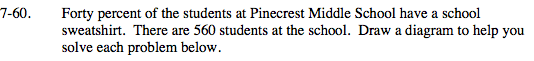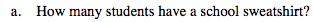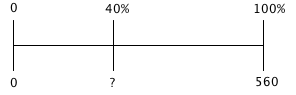$\frac{40}{100}\left(\frac{?}{?}\right)=\frac{\textit{x}}{560}$

x = 224 students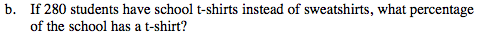280 is what percent of 560?

$\frac{\textit{x}}{100}\left(\frac{?}{?}\right)=\frac{280}{560}$

x = 50%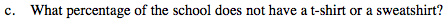If 40% of the students have a school sweatshirt and 50% have a t-shirt instead of a sweatshirt, what is the remaining percentage of students that have neither?